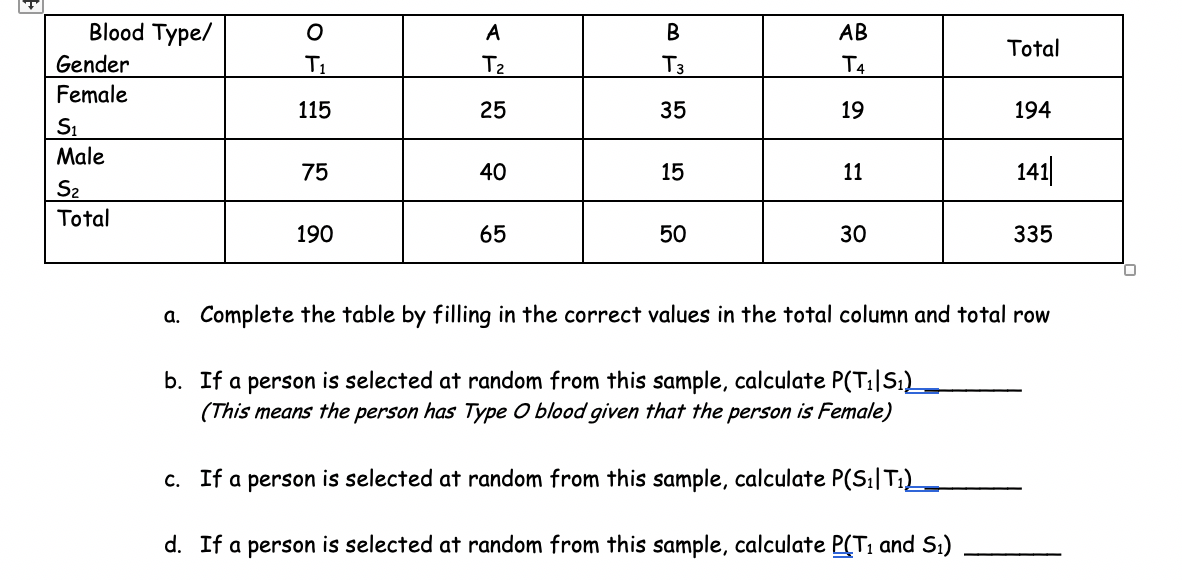# Blood Type/ о В АВ Total Gender T1 T2 Тз T4 Female 115 25 35 19 194 S1 Male 141 75 40 15 11 S2 Total 190 65 50 30 335 a. Complete the table by filling in the correct values in the total column and total row b. If a person is selected at random from this sample, calculate P(T1IS1) (This means the person has Type O blood given that the person is Female) c. If a person is selected at random from this sample, calculate P(S1T1) d. If a person is selected at random from this sample, calculate P(T1 and S.)

Question

I need help understanding how to solve b-d. I'm confused as to how they equal different solutions.help_outlineImage TranscriptioncloseBlood Type/ о В АВ Total Gender T1 T2 Тз T4 Female 115 25 35 19 194 S1 Male 141 75 40 15 11 S2 Total 190 65 50 30 335 a. Complete the table by filling in the correct values in the total column and total row b. If a person is selected at random from this sample, calculate P(T1IS1) (This means the person has Type O blood given that the person is Female) c. If a person is selected at random from this sample, calculate P(S1T1) d. If a person is selected at random from this sample, calculate P(T1 and S.) fullscreen

## Expert Answer

1 Rating

### Want to see this answer and more?

Experts are waiting 24/7 to provide step-by-step solutions in as fast as 30 minutes!*

*Response times vary by subject and question complexity. Median response time is 34 minutes and may be longer for new subjects.
Tagged in
MathStatistics

### Other© 2021 bartleby. All Rights Reserved.